# Sum of First N Natural Number in C

## Sum of First N Natural Number in C Language:

In this article, I am going to discuss the sum of first n natural number by using the following ways:

1. Direct Function
2. Loop
3. Recursion

Let’s discuss each way with a detailed explanation.

##### Sum of First N Natural Number by using Direct Function:

In the below example, we will find the sum of the first ‘N’ natural number by using a simple formula n * (n + 1) / 2. We first created a function of name sum which takes only one parameter which holds the value of n. In this function, we simply return the sum by using the above formula, and then in the main function, we print that value on the screen.

```#include <stdio.h>
int sum(int v) {
return v * (v + 1) / 2;
}

int main() {
int v = 6;
printf("%d", sum(v));
}
```

Output: 21

Time Complexity: O(1)

##### Sum of First N Natural Number by using Loop:

In the below example, we will find the sum by using for loop. In the main function, first, we declare two variables v and sum (value of zero). On the next line, we give some value to variable v which will find the sum up to that value. Then, we run our for loop and inside this loop, we add a statement “sum = sum + i” which modifies our sum variable. And the last statement is the print statement which prints our sum on the screen.

```#include <stdio.h>
int main() {
int v, sum = 0;
v = 6;

for (int i = 1; i <= v; i++) {
sum = sum + i;
}
printf("%d", sum);
}
```

Output: 21

Time Complexity: O(n)

##### Sum of First N Natural Number by using Recursion:

We can also find the sum of n natural numbers with recursion. Let’s understand each and every step. In the below example, we created a function sum that takes one parameter. In the next step, we define our base condition (where the function terminates or ends) which is if v == 0. If it satisfies then return 0 otherwise return sum (v – 1) + v.

```#include <stdio.h>
int sum(int v) {
if (v == 0)
return 0;
return sum(v - 1) + v;
}

int main() {
int v = 6;
printf("%d", sum(v));
}
```

Time Complexity: O(n)

Output: 21

##### Tracing Tree of the above Recursion Program:

Now, Let’s jump on the Tracing Tree where we will understand each and every step.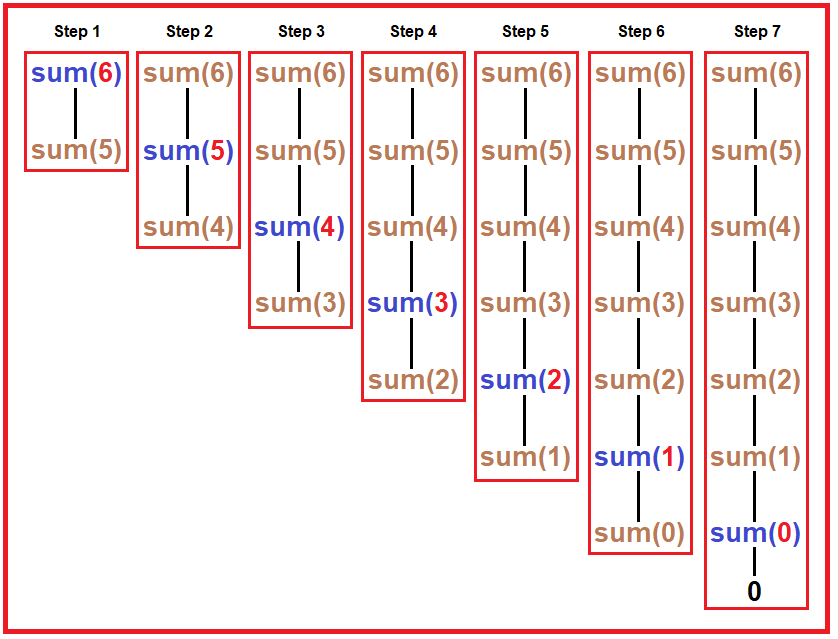##### Step1.

Tracing Tree: In the above diagram, there are 7 steps. In every step, we have shown every recursive call of the sum function. We passed 6 as a parameter.

1. In 1st step, it checks if 6 == 0? No, then it goes to the else part which returns sum (6 – 1) + 6. Here we can’t perform addition, first, it has to finish sum (6 – 1) call then only addition will perform.
2. In 2nd step, it checks if 5 == 0? No, then it goes to the else part which returns the sum (5 – 1) + 5. Here also we will have to first calculate the result of sum (5 – 1) call then only addition will perform.
3. In 3rd step, it checks if 4 == 0? No, then it goes to the else part which returns the sum (4 – 1) + 4. we will have to first calculate the result of the sum (4 – 1) call then only the addition will perform.
4. In 4th step, it checks if 3 == 0? No, then it goes to the else part which returns the sum (3 – 1) + 3. we will have to first calculate the result of the sum (3 – 1) call then only the addition will perform.
5. In 5th step, it checks if 2 == 0? No, then it goes to the else part which returns the sum (2 – 1) + 2. we will have to first calculate the result of the sum (2 – 1) call then only the addition will perform.
6. In 6th step, it checks if 1 == 0? No, then it goes to the else part which returns the sum (1 – 1) + 1. we will have to first calculate the result of the sum (1 – 1) call then only the addition will perform.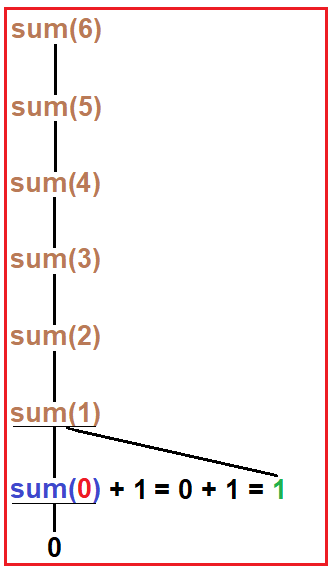##### Step2:

Tracing Tree: Now, here sum (0) checks for the condition if 0 == 0? Yes, then it returns 0. And this 0 is return inside the function call sum (1). Now addition takes place as 0 + 1 = 1 and now this 1 is return from sum (1) to sum (2).

Note: The addition will take place on the returning time from the recursive calls.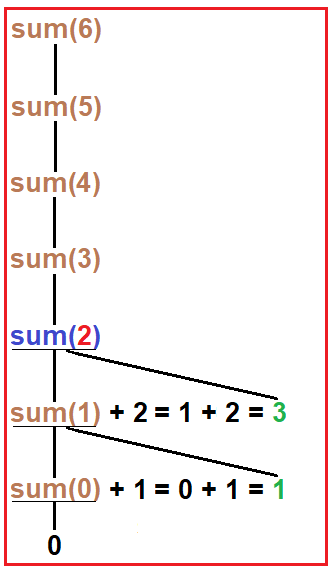##### Step3:

Tracing Tree: As we discussed in the previous step that sum (1) returns 1 to sum (2) call. Now addition takes place as 1 + 2 = 3 and now this 3 is returning from sum (2) to sum (3).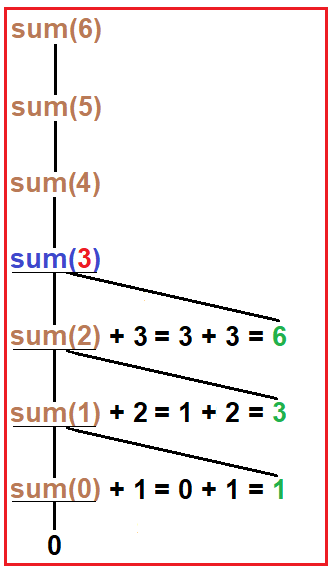##### Step4:

Tracing Tree: Now, 3 was returning to sum (3). Here addition takes place as 3 + 3 = 6 and then 6 will return to sum (4) and in sum (4), addition will take place as 6 + 4 = 10 and then 10 will return to sum (5) where addition takes place as 10 + 5 = 15 and then it returns to sum (6).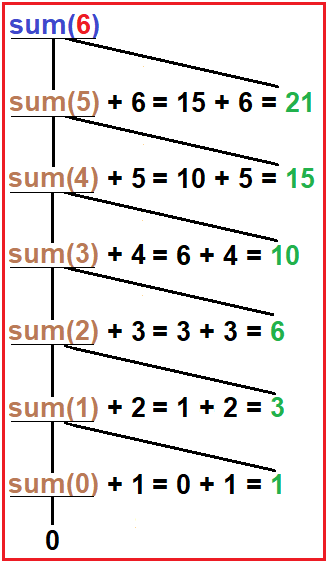##### Step5:

Tracing Tree: As discussed in the previous step, 15 was returned to sum (6) and here addition takes place as 15 + 6 = 21 and now all recursive calls have finished and then we print 21 on the output screen.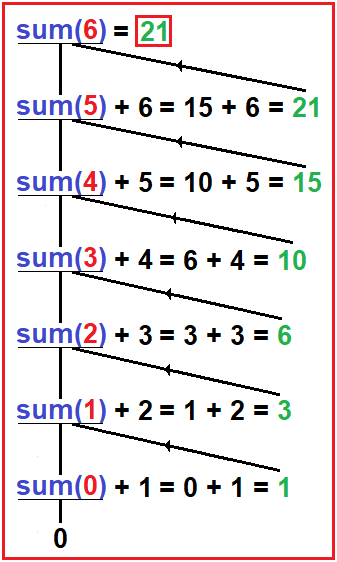In the next article, I am going to discuss Factorial of a number in C Language with Examples. Here, in this article, I try to explain the sum of the first N natural number in C Language with Example and I hope you enjoy this sum of the first N natural number in C Language with Example article.